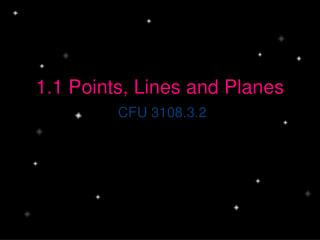DownloadDownload Presentation1.1 Points, Lines and Planes

# 1.1 Points, Lines and Planes

Télécharger la présentation## 1.1 Points, Lines and Planes

- - - - - - - - - - - - - - - - - - - - - - - - - - - E N D - - - - - - - - - - - - - - - - - - - - - - - - - - -
##### Presentation Transcript

1. 1.1 Points, Lines and Planes CFU 3108.3.2

2. Remember you have 5 minutes to get started. Chapter 1Define and place in your glossary. • Line • Line segment • Point • Plane • Congruent • Segment bisector • Collinear • Coplanar • Precision • Accuracy • Ray • Angle bisector • Perpendicular • Complementary Angles • Supplementary angles • Intersection • Polygon • Concave • Convex • Regular Polygon • Polyhedron • Vertex

3. Objective and Standard • Introduce proper conventions for naming points, lines and planes and identifying intersections of lines and planes. • Recognize that a definition depends on undefined terms and on previous definitions. • Describe the intersection of lines (in the plane and in space), a line and a plane, or of two planes. • Develop the structures of geometry, such as lines, angles, planes, and planar figures, and explore their properties and relationships. • Give precise mathematical descriptions or definitions of geometric shapes in the plane and space.

4. Key Points n B Y T A X Z

5. Name Line & Plan l E A B C D N List two points to name line l AB, BA, AC, CA, AD, DA, BC, CB, BD, DB, CD, DC List three point names for plane N ABE, ACE, ADE, BCE, BDE, CDE

6. Draw Geometric Figures • Lines GH and JK intersect at L for • G(-1, -3), H(2,3), J(-3, 2) & K(2, -3) • Point M is coplanar with these points but not collinear with GH or JK. H J M L K G • Graph GH and JK and M • Label the Intersection L

7. Interpret Drawing • How many planes in the figure? • 4 • Name three points that are collinear • D, B, G • Are points G,A,B and E collinear? • At what point do EF and AB intersect? F D P G C E B A

8. Summarize Objective: Introduce proper conventions for naming points, lines and planes and identifying intersections of lines and planes

9. Assignment – Block Class • Page 8, 14 - 46 even and 62, 64 • Page 9, 32 - 78 Even Assignment – Honors Class